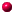What is this thing called Painlevé? If you are interested in the field of non-linear differential equations and how to identify the ones that have no chaos whatsover, you need to know what the adjective Painlevé means. The purpose of this page is to give you a gentle introduction to this exploding field. There are six classical second-order non-linear differential equations that are called the Painlevé equations: $$\begin{eqnarray*} y''&=&6y^2+x\\ y''&=&2y^3+xy+\alpha\\ y''&=&\frac{{y'}^2}{y}-\frac{y}{x}+\frac{1}{x}(\alpha y^2+\beta) +\gamma y^3+\frac{\delta}{y}\\ y''&=&\frac{{y'}^2}{2y}+\frac{3 y^3}{2}+4xy^2+2(x^2-\alpha)y +\frac{\beta}{y}\\ y''&=&\Biggl(\frac{1}{2y}+\frac{1}{y-1}\Biggr){y'}^2-\frac{y'}{x} +\frac{(y-1)^2}{x^2 y}(\alpha y^2+\beta) +\frac{\gamma y}{x} +\frac{\delta y(y+1)}{y-1} \\ y''&=&\frac{1}{2}\Biggl(\frac{1}{y}+\frac{1}{y-1}+\frac{1}{y-x}\Biggr){y'}^2 -\Biggl(\frac{1}{x}+\frac{1}{x-1}+\frac{1}{y-x}\Biggr)y'\\ &&\quad\quad+\frac{y(y-1)(y-x)}{x^2(x-1)^2}\Biggl(\alpha+ \frac{\beta x}{y^2} +\frac{\gamma (x-1)}{(y-1)^2}+\frac{\delta x(x-1)}{ (y-x)^2}\Biggr) \end{eqnarray*}$$ In these equations, $$y$$ is a function of $$x$$, primes denote differentiation with respect to $$x$$ and $$\alpha$$, $$\beta$$, $$\gamma$$, $$\delta$$ denote parameters. The solutions of these equations have amazing properties. To find out more, you will need to read the pages below. CONTENTS: 0. ODEs and Singularities 0.0. Introductory examples 0.1. Ordinary and Singular Points 0.2. Regular Singular Points and Movable Singularities 1. Discrete and Difference Equations 1.0. Introductory examplesHome Page The contents will be expanded gradually. If there is something you are dying to find out, email me. Last modified: 15 October 2013 by N.Joshi